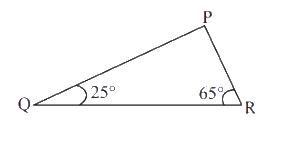# Ex.6.5 Q6 The Triangle and Its Properties - NCERT Maths Class 7

Go back to  'Ex.6.5'

## Question

Angles $$Q$$ and $$R$$ of a $$\Delta\,PQR$$ are $$25^\circ$$ and $$65^\circ$$Write which of the following is true:

(i) $$PQ^2 + QR^2 = RP^2$$

(ii) $$PQ^2 + RP^2 = QR^2$$

(iii) $$RP^2 + QR^2 = PQ^2$$Video Solution
Triangle & Its Properties
Ex 6.5 | Question 6

## Text Solution

What is known?

Measure of two angles of a triangle i.e. $$25°$$and $$65°.$$

What is the unknown?

Which option is correct.

Reasoning:

This question is straight forward, in this question it is clear from the figure two angles of a triangle are given and we must find out the third angle by using angle sum property i.e. the sum of three interior angles of a triangle is $$180^\circ$$ By using this property, we got the value of third angle i.e. $$P = 90^\circ$$ that means side opposite to $$P$$ is hypotenuse i.e. $$QR$$. As one of the angles is $$90^\circ$$ that means it is a right-angled triangle and the square of hypotenuse is equal to the sum of square of other two sides.

Steps:

We know that, sum of interior angles of a triangle is $$180^\circ$$.

\begin{align}\angle P + \angle Q + \angle R &= 180^\circ \\\angle P + 25^\circ  + 65^\circ  &= 180^\circ \\\angle P + 90^\circ  &= 180^\circ \\\angle P &= 180^\circ  - 90^\circ \\\angle P &= 90^\circ \end{align}

Thus, triangle $$PQR$$ is a right angled at $$P$$

Therefore, by Pythagoras theorem

\begin{align}{(P)^2} + {(B)^2} &= {(H)^2}\\{(QP)^2} + {(PR)^2} &= {(QR)^2}\end{align}

Hence, option (ii) is correct.

Useful Tip:

Whenever you encounter problem of this kind, it is best to think of the Pythagoras property if one of the three angles is $$90^\circ$$ then the square of hypotenuse or greater side is equal to the sum of square of other two sides.

Learn from the best math teachers and top your exams

• Live one on one classroom and doubt clearing
• Practice worksheets in and after class for conceptual clarity
• Personalized curriculum to keep up with school